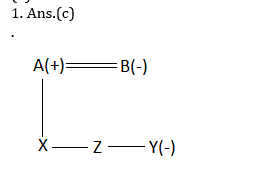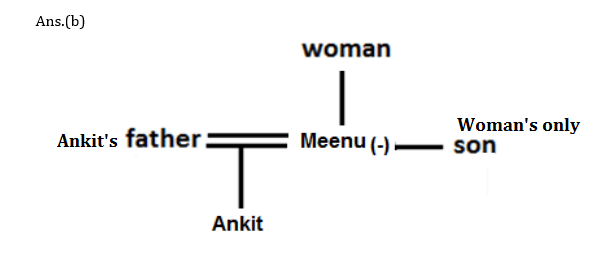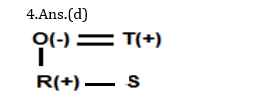# Blood relation for IBPS, SBI, RBI, RRBs: Quiz 3

## Blood relation for IBPS, SBI, RBI, RRBs: Quiz 3

Blood relation plays a significant role in Reasoning Ability Section of banking exams such as IBPS, SBI and RBI PO and Clerk. You will get at least 5 questions from Blood relation in one of IBPS, SBI and RBI PO & clerk prelims and Mains exam. So, aspirants should focus on Blood relation questions in detail. Here, we are providing you with the Blood relation questions quiz with the detailed solution so that you can easily prepare for Blood relation questions. We are providing here all-important latest pattern-based questions and Previous Year Questions of Blood relation of various Government Exam like IBPS, SBI, and RBI PO and Clerk exam. This Blood relation quiz we are providing is free. Attempt this Blood relation quiz to practice important questions with answers and solutions. And score better in IBPS, SBI and RBI PO and Clerk exam.

Blood relation Quiz to improve your Reasoning Ability for SBI Po & SBI clerk exam IBPS PO Reasoning, IBPS Clerk Reasoning, IBPS RRB Reasoning, LIC AAO, LIC Assistant and another competitive exam.

Q1. A is father of X; B is mother of Y. The sister of X and Z is Y. Which of the following statements is definitely not true?

(a) B is the mother of Z

(b) X is the sister of Z

(c) Y is the son of A

(d) B has one daughter

(e) B is the wife of A

Answer & ExplanationQ2. Pointing to awoman, Meenu says to Ankit,“she is my mother and her only son is your maternal uncle.” In this relation Meenu is Female. How is the narrator related to Ankit’s father?

(a) Brother

(b)Wife

(c)Sister

(d)Mother

(e)Father

Answer & ExplanationDirections (3-5): Study the following information carefully and answer the questions that follow.

‘A \$ B’ means ‘A is father of B’.

‘A # B’ means ‘A is mother of B’.

‘A @ B’ means ‘A is wife of B’.

`A % B’ means ‘A is son of B’.

Q3. Which of the following expressions indicates ‘T is mother of Q?

(a) T@C%R\$Q

(b) T#B%V\$Q@M

(c) Q%C@R\$T

(d) V\$Q@R\$T

(e) None of these

Answer & ExplanationQ4. If the expression is O #R % T \$ S, then how is T related to R?

(a) Brother-in-law

(b) Sister-in-law

(c) Brother

(d) Father

(e) None of these

Answer & ExplanationQ5. If the expression is D@Q\$N@P%M, then how is M related to N?

(a) Mother

(b) Sister

(c) Brother

(d) Father

(e) Can’t be determined.

Answer & ExplanationDirections (6-7): Study the information carefully and answer the questions given below.

M, N, O, P , Q and R are six family members. Q is son of P, who is not the mother of Q. N is brother of P. R and P are a married couple. O is daughter of R, who is sister of M.

Q6. How many female members are there in the family?

(a) Two

(b) Three

(c) Four

(d) Can’t be determined

(e) None of these

Answer & Explanation

1. Ans.(d)

Q7. How is Q related to O?

(a) Mother

(b) Father

(c) Sister

(d) Brother

(e) None of these

Answer & Explanation

1. Ans.(d)
1. If A + B means A is the brother of B; A – B means A is the sister of B and A x B means A is the father of B. Which of the following means that C is the son of M?

(A) M – N x C + F

(B) F – C + N x M

(C) N + M – F x C

(D) M x N – C + F

(E)None

Answer & Explanation

1. Ans(d)
• Pointing to a girl Sandeep said, “She is the daughter of the only sister of my father.” How is sandeep related to the girl?
• (A) Uncle(B) Cousin

(C) Father

(D) Grandfather

(E)Son

Answer & Explanation
9. Ans(b)

10.A is the brother of B. B is the brother of C. D is the father of A. Based on these three statements, which of the following statements cannot be definitely true?

(A) A is the brother of C

(B) C is the brother of A

(C) B is the brother of A

(D) B is the son of D

(E) A , B and C are D’s children

Answer & Explanation
10. Ans(b)
• Online Mock Test Available on App as well as Web:

##### 2020 Preparation Kit PDF

#### Most important PDF’s for Bank, SSC, Railway and Other Government Exam : Download PDF Now

The Banking Awareness 500 MCQs E-book| Bilingual (Hindi + English) Get PDF here
AATMA-NIRBHAR Series- Banking Awareness Practice Ebook PDF Get PDF here
Computer Awareness Capsule 2.O Get PDF here
AATMA-NIRBHAR Series Quantitative Aptitude Topic-Wise PDF 2020 Get PDF here
AATMA-NIRBHAR Series- Static  Awareness Practice Ebook PDF Click Here
Memory Based Puzzle E-book | 2016-19 Exams Covered Get PDF here
Caselet Data Interpretation 200 Questions Get PDF here
Puzzle & Seating Arrangement E-Book for BANK PO MAINS (Vol-1) Get PDF here
ARITHMETIC DATA INTERPRETATION 2.O E-book Get PDF here